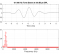## Problem with 1st order Massberg LPFStarted by 5 years ago3 replieslatest reply 5 years ago123 views

I'm trying to implement this filter in title by following the book "Designing Audio Effect Plug-Ins in C++" By Will Pirkle

Problem I'm facing is the magnitude response error when comparing against analog model of the LP filter. Here is a plot showing the difference:I get these are values for variables (fc=1000, fs=44100):

>> massberg_test

w0 =  0.14248
g1 =  0.045305
gm =  0.70711
wm =  6283.2
Om =  0.071359
Os =  0.10081
g0 =  1.1008
a0 =  0.14612
a1 =  0.055508
b1 = -0.89919
Real:
a = [ 0.132736  0.050424 ]
b = [ 1.00000  -0.81684 ]

I don't have access to the original paper by M. Massberg so, I can't be sure if the equations in my source book is correct (I've read that the "ac" in g1 formula is just a typo and book errata does not mention other errors for this fileter).

Q:

1. Are the equations found in Pirkle book correct?

2. If 1 is Yes then where to look the issue from?

[ - ]I think the value for Omega_s is not correct.  The value that I get is 0.07121.  Since the "b0", "b1", "a0", and "a1" coefficients are computed using Omega_s then they are incorrect too.

Using the equations in the body of the text from the Massberg (2011) paper,

fc=1e3;
Fs=44.1e3;

Wn=2*pi*fc/Fs;

g1=2/sqrt(4+(Fs/fc)^2);  % Okay
gm=max(sqrt(0.5), sqrt(g1));  % Okay
wm=2*pi*fc*sqrt(1-gm^2)/gm;  % Okay

Om=tan(wm/(2*Fs));  % Okay
Os=Om*sqrt((gm^2-g1^2)*(1-gm^2))/(1-gm^2);  % Check

b0=Os+g1; b1=Os-g1;
a0=Os+1; a1=Os-1;

b=[b0/a0 b1/a0];
a=[1 a1/a0];
figure; freqz(b, a, 4096, Fs);

[bf, af] = lp1(Wn);
figure; freqz(bf, af, 4096, Fs);

The Matlab code from the Massberg (2011) paper for the first-order lowpass filter is,

function [b,a] = lp1(Wn)

g1 = 2/sqrt(4+(2*pi/Wn)^2);
gm = max(sqrt(0.5),sqrt(g1));
Ws = tan(Wn*sqrt(1-gm^2)/(2*gm)) *sqrt((gm^2-g1^2)*(1-gm^2)) /(1-gm^2);
b0 = Ws+g1;
b1 = Ws-g1;
a0 = Ws+1;
a1 = Ws-1;
b = [b0/a0 b1/a0];
a = [1 a1/a0];

where Wn is,

Wn=2*pi*fc/Fs;
[ - ]